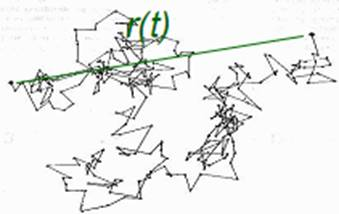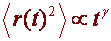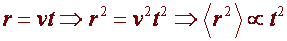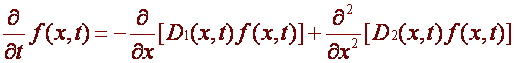FORWARD

Random Walks and Plasmas

We have described two fields that seem to have nothing to do with each other. However this is far from being true. The topic of this last section will be to show how random walks can prove useful in Plasma Physics. We should warn the reader that this is a rather technical matter, so we will try only to sketch some basic features of it.Let’s suppose that the starting point and the ending point of a random walk are separated by a distance r(t). We now consider the mean square displacement, which is proportional to some power of the lapsed time t, that is.

Anomalous diffusion is characterized by the exponent γ, which define the nature of the walk as follows

·                   γ=1: classical diffusion

·                   γ>1: super-diffusion (in the special case γ=2, ballistic diffusion)

·                   γ<1:  sub-diffusion

Anomalous diffusion can lead to statistical properties of plasmas, such as density and temperature profiles exhibiting anomalous (which means strange or unexpected) behavior.

Some examples effects caused by anomalous diffusion:

·                   Profile stiffness, meaning that the density or temperature profile stays close to a certain shape, even if the plasma is distorted

·                   Cooling at the edge can lead to a temperature rise at the center

·                   During heating at the edge, there can be an inward electron heat flux, in direction of increasing temperature (so-called “uphill” transport of energy

·                   Sudden cooling at the edge may lead to a temperature drop that propagates inwards with constant velocity (, super-diffusion)

In a turbulent plasma, a particle can freely travel along the magnetic field lines, drift across the magnetic field lines, be locally trapped or locally accelerated.

Turbulence implies complex spatiotemporal structures, where each individual particle performs a random walk!

So does it work?

YES! It has been shown that Random Walk can successfully model observed phenomena of anomalous transport in confined plasmas, such as profile stiffness and uphill transport.

The final food for thought.

Uses of random in describing the above phenomena can be found in the following papers

Montroll, E.W. Weiss, G.H, J. Math. Phys. 6, 167 (1965)

Balescu, R., Phys. Rev. E 51, 4807 (1995)

Van Milligen, B.Ph., Sanchez, R., Carreras, B.A., PoP 11, 2272 (2004)

Van Milligen, Carreras, B.A., B.Ph., Sanchez, R., PoP 11, 3787 (2004)

Finally, we must mention the Fokker-Planck equation, which follows from Browninan motion and was first used to describe the motion of a particle in a fluid (also a plasma). The Fokker Planck equation is the major link between plasmas and random walks, however we just mention it now, since we wanted to present some new developments in the subject.

Without proving it, the 1-D Fokker Planck equation for some process f(x,t) with drift D1(x,t) and diffusion D2(x,t) isTHE END